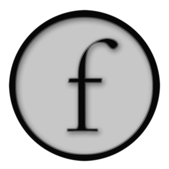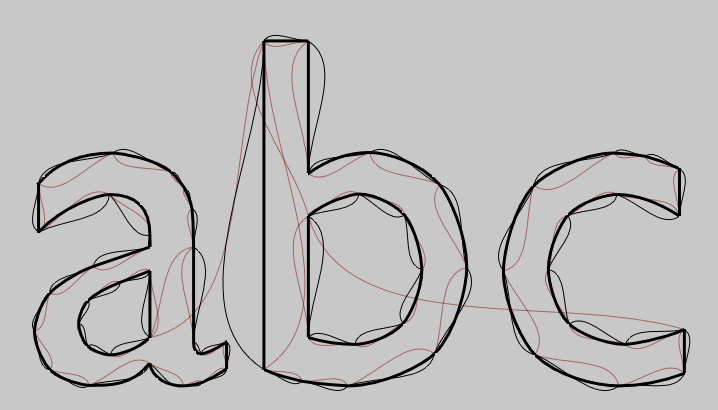FLine Node reference

`FLine` geometry containers are made up of control nodes. Roughly speaking, you get one control node for every `.moveTo(...)`, `.lineTo(...)`, or `.cubicTo(...)` you issue. Nodes have positions: 1 in the case of `.moveTo` or `.lineTo` or 3 in the case of `.cubicTo`. This code illustrates:

``````f = FLine().moveTo(10,10).lineTo(30,40).cubicTo(60,30,100,80, 130, 40).lineTo(160,50)
for n in f.nodes:
dots = FLine(pointed=1, pointSize=10, thickness=3, color=Color4(0.5, 0, 0, 0.4))
for q in n.points():
dots.lineTo(*q)
``````

This results in four nodes `.moveTo()`, `.lineTo()`, `.cubicTo()`, `.lineTo()`As you can see from the code above you can access, inspect and modify the nodes of an `FLine` by accessing elements of the `.nodes` array. The page collects useful methods that you can perform on a node (an instance of `CachedLine.Event`), but as always, you can get the most complete, most up-to-date list through autocompletion.

`.arbitraryProperty_v="peach"`

You can set and get arbitrary properties on nodes. By convention they have the `_v` suffix (since this is what `FLine` uses to indicate that you want to set a property on the most recently created node). Some properties are handled as special signals to Field to do interesting things (change point styles, draw text etc...) take a look at the list.

`node +# CFrame(r0.2)`

You can transform nodes using `CFrame` instances, as well as `Quaternion`s and `Vector3` and `Vector2` (see SimpleLinearAlgebra for an overview of this zoo). Transforming nodes and setting properties on them accounts for basically everything you want to do at this level with lines. Some examples:

``````f = FLine()
for n in floatRange(0, 10, 10):
f.cubicTo(n*14, n*20, n*20, n*14, n*20, n*20)

f = f.copy()
f.color=Color4(0.5, 0, 0, 1.0)
for n in range(len(f.nodes)):
f.nodes[n] += Vector2(n*10,0)

f = f.copy()
f.color=Color4(0, 0, 0.5, 1.0)
for n in range(len(f.nodes)):
f.nodes[n] += Quaternion(-n*0.04)
f.nodes[n] += Vector2(-n*4,0)
```````CFrame` also accepts `FLine` nodes for transform centers, which yields this pattern here (to have rotations and scales centered on the "head" of the line, see below):

``````for t in range(0, 20):
f = f.copy()
for n in f.nodes:
```````.setPosition(x,y[,z])`

Gets or sets the position (end-point) of this node. This is a `Vector3` (see SimpleLinearAlgebra for more). If you really want a `Vector2` use `.position2()`.

`.isCubic()`

Returns `1` if this is a cubic spline segment. Otherwise, returns 0.

`.isConnected()`

Returns `1` if this is a cubic spline segment or a line. Otherwise, returns 0.

`.control1()`, `.setControl1(x,y[,z])`, `.control2()`, `.setControl2(x,y[,z])`

Gets or sets the first or second control points of a node if it has them.

`.points()`, `.setPoints([c1,c2,],p)`

Gets or sets all the points (all the `Vector3` positions) in this node. Either 1 (for `.moveTo` or `.lineTo`) or 3 (`.cubicTo`).

`.next()`, `.previous()`, `.head()`

These three functions navigate between nodes, `next()` and `previous()` are self explanatory — the nodes are a list, these return the previous and the next node in the list. `head()` goes backwards but only if this node is actually connected to the previous node, otherwise it returns the current node. Thus `.head()` of a `.moveTo()` is that `.moveTo()` not some potentially unrelated previous node.

This code and image illustrate the difference:

``````_self.lines.clear()
f = FLine().appendVectorText2("abc", 0, 0, size=500)
f.thickness=3

for n in f.nodes: